The Markov process is one of the part of stochastic process in which it is necessary for us to satisfy a Markovian equation:

P {Xt + 1 = j | X0 = k0, X1 = k1… , Xt-1 = k t-1 ,X t= i}

= P {X t+1  = j | Xt = i} for t = 0, 1, …

This equation shows that the environment that is going to occur in future depends directly and only on the environment of the next day.

There are two of transition probability:

• First one is known as one step transition probability which is given by the equationP { Xt+1  = j | Xt = i} which shows the process between time period t and t+1.

Further a one-step conditional probability is divided into stationary and non-stationary probabilities. The stationary one-step conditional probability is shown by the equation:

P {X t+1  = j | Xt = i} = P {X1 =j | X0 = i} for all t = 0, 1, …

Where transition remain fixed with time. This equation is denoted by Pij.

• Another type is known as n-step transition probability which is shown by the equation:

P {Xt+n=j | X, = i} = P {Xn = j | X0= i} for all t = 0, 1, ..,

And is denoted by pij(n) for all i and j.

Both the equation will further satisfy two important properties:

• pij(n )≥ 0 for all i and j  and n = 0, 1, 2, …
• ∑ pij(n )=1 for all i andn = 0, 1, 2, … , and  M =No. of states.

Matrix form of transition probabilities:

Transition of conditional probabilities can also be represented in matrix form.

1. N-step transition probabilities:
1. One-step transition probabilities:

Thus, we see from the above matrix forms that P denotes one-step transition probabilities and P(n)  is called n-step transition probabilities.

Finite state Markov chain:

A countable number of forms, the Markovian property, stationary transition probabilities and the set of probabilities P {X0 = i} together satisfy the finite state of Markov theorem of a stochastic process {X1} (t=0,1,2,…).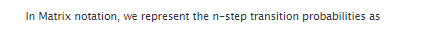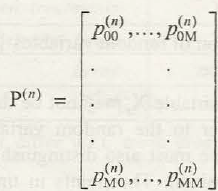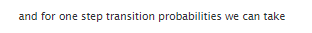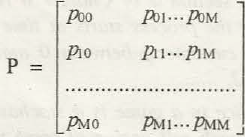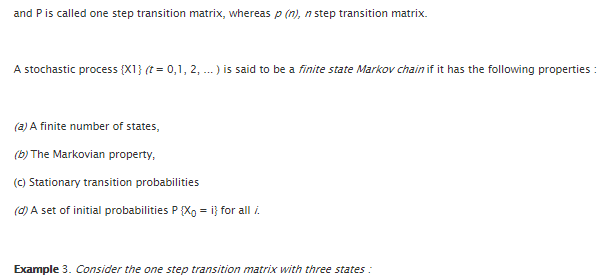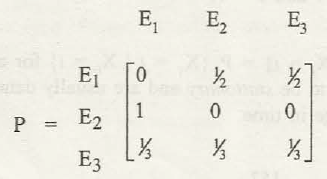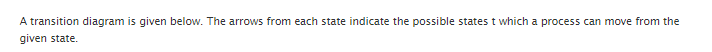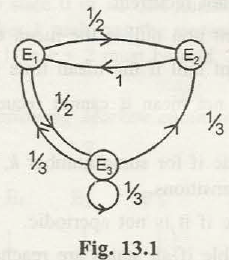### Customer Reviews

My Homework Help
Rated 5.0 out of 5 based on 510 customer reviews at